Code examples / Computer Vision / Focal Modulation: A replacement for Self-Attention

# Focal Modulation: A replacement for Self-Attention

Author: Aritra Roy Gosthipaty, Ritwik Raha
Date created: 2023/01/25
Description: Image classification with Focal Modulation Networks.

ⓘ This example uses Keras 2View in ColabGitHub source

## Introduction

This tutorial aims to provide a comprehensive guide to the implementation of Focal Modulation Networks, as presented in Yang et al..

This tutorial will provide a formal, minimalistic approach to implementing Focal Modulation Networks and explore its potential applications in the field of Deep Learning.

Problem statement

The Transformer architecture (Vaswani et al.), which has become the de facto standard in most Natural Language Processing tasks, has also been applied to the field of computer vision, e.g. Vision Transformers (Dosovitskiy et al.).

In Transformers, the self-attention (SA) is arguably the key to its success which enables input-dependent global interactions, in contrast to convolution operation which constraints interactions in a local region with a shared kernel.

The Attention module is mathematically written as shown in Equation 1.Equation 1: The mathematical equation of attention (Source: Aritra and Ritwik)

Where:

• `Q` is the query
• `K` is the key
• `V` is the value
• `d_k` is the dimension of the key

With self-attention, the query, key, and value are all sourced from the input sequence. Let us rewrite the attention equation for self-attention as shown in Equation 2.Equation 2: The mathematical equation of self-attention (Source: Aritra and Ritwik)

Upon looking at the equation of self-attention, we see that it is a quadratic equation. Therefore, as the number of tokens increase, so does the computation time (cost too). To mitigate this problem and make Transformers more interpretable, Yang et al. have tried to replace the Self-Attention module with better components.

The Solution

Yang et al. introduce the Focal Modulation layer to serve as a seamless replacement for the Self-Attention Layer. The layer boasts high interpretability, making it a valuable tool for Deep Learning practitioners.

In this tutorial, we will delve into the practical application of this layer by training the entire model on the CIFAR-10 dataset and visually interpreting the layer's performance.

Note: We try to align our implementation with the official implementation.

## Setup and Imports

We use tensorflow version `2.11.0` for this tutorial.

``````import numpy as np
import tensorflow as tf
from tensorflow import keras
from tensorflow.keras import layers
from typing import Optional, Tuple, List
from matplotlib import pyplot as plt
from random import randint

# Set seed for reproducibility.
tf.keras.utils.set_random_seed(42)
``````

## Global Configuration

We do not have any strong rationale behind choosing these hyperparameters. Please feel free to change the configuration and train the model.

``````# DATA
TRAIN_SLICE = 40000
BUFFER_SIZE = 2048
BATCH_SIZE = 1024
AUTO = tf.data.AUTOTUNE
INPUT_SHAPE = (32, 32, 3)
IMAGE_SIZE = 48
NUM_CLASSES = 10

# OPTIMIZER
LEARNING_RATE = 1e-4
WEIGHT_DECAY = 1e-4

# TRAINING
EPOCHS = 25
``````

## Load and process the CIFAR-10 dataset

``````(x_train, y_train), (x_test, y_test) = keras.datasets.cifar10.load_data()
(x_train, y_train), (x_val, y_val) = (
(x_train[:TRAIN_SLICE], y_train[:TRAIN_SLICE]),
(x_train[TRAIN_SLICE:], y_train[TRAIN_SLICE:]),
)
``````
``````Downloading data from https://www.cs.toronto.edu/~kriz/cifar-10-python.tar.gz
170498071/170498071 [==============================] - 30s 0us/step
``````

### Build the augmentations

We use the `keras.Sequential` API to compose all the individual augmentation steps into one API.

``````# Build the `train` augmentation pipeline.
train_aug = keras.Sequential(
[
layers.Rescaling(1 / 255.0),
layers.Resizing(INPUT_SHAPE + 20, INPUT_SHAPE + 20),
layers.RandomCrop(IMAGE_SIZE, IMAGE_SIZE),
layers.RandomFlip("horizontal"),
],
name="train_data_augmentation",
)

# Build the `val` and `test` data pipeline.
test_aug = keras.Sequential(
[
layers.Rescaling(1 / 255.0),
layers.Resizing(IMAGE_SIZE, IMAGE_SIZE),
],
name="test_data_augmentation",
)
``````

### Build `tf.data` pipeline

``````train_ds = tf.data.Dataset.from_tensor_slices((x_train, y_train))
train_ds = (
train_ds.map(
lambda image, label: (train_aug(image), label), num_parallel_calls=AUTO
)
.shuffle(BUFFER_SIZE)
.batch(BATCH_SIZE)
.prefetch(AUTO)
)

val_ds = tf.data.Dataset.from_tensor_slices((x_val, y_val))
val_ds = (
val_ds.map(lambda image, label: (test_aug(image), label), num_parallel_calls=AUTO)
.batch(BATCH_SIZE)
.prefetch(AUTO)
)

test_ds = tf.data.Dataset.from_tensor_slices((x_test, y_test))
test_ds = (
test_ds.map(lambda image, label: (test_aug(image), label), num_parallel_calls=AUTO)
.batch(BATCH_SIZE)
.prefetch(AUTO)
)
``````
``````WARNING:tensorflow:From /usr/local/lib/python3.8/dist-packages/tensorflow/python/autograph/pyct/static_analysis/liveness.py:83: Analyzer.lamba_check (from tensorflow.python.autograph.pyct.static_analysis.liveness) is deprecated and will be removed after 2023-09-23.
Instructions for updating:
Lambda fuctions will be no more assumed to be used in the statement where they are used, or at least in the same block. https://github.com/tensorflow/tensorflow/issues/56089
``````

## Architecture

We pause here to take a quick look at the Architecture of the Focal Modulation Network. Figure 1 shows how every individual layer is compiled into a single model. This gives us a bird's eye view of the entire architecture.Figure 1: A diagram of the Focal Modulation model (Source: Aritra and Ritwik)

We dive deep into each of these layers in the following sections. This is the order we will follow:

• Patch Embedding Layer
• Focal Modulation Block
• Multi-Layer Perceptron
• Focal Modulation Layer
• Hierarchical Contextualization
• Gated Aggregation
• Building Focal Modulation Block
• Building the Basic Layer

To better understand the architecture in a format we are well versed in, let us see how the Focal Modulation Network would look when drawn like a Transformer architecture.

Figure 2 shows the encoder layer of a traditional Transformer architecture where Self Attention is replaced with the Focal Modulation layer.

The blue blocks represent the Focal Modulation block. A stack of these blocks builds a single Basic Layer. The green blocks represent the Focal Modulation layer.Figure 2: The Entire Architecture (Source: Aritra and Ritwik)

## Patch Embedding Layer

The patch embedding layer is used to patchify the input images and project them into a latent space. This layer is also used as the down-sampling layer in the architecture.

``````class PatchEmbed(layers.Layer):
"""Image patch embedding layer, also acts as the down-sampling layer.

Args:
image_size (Tuple[int]): Input image resolution.
patch_size (Tuple[int]): Patch spatial resolution.
embed_dim (int): Embedding dimension.
"""

def __init__(
self,
image_size: Tuple[int] = (224, 224),
patch_size: Tuple[int] = (4, 4),
embed_dim: int = 96,
**kwargs,
):
super().__init__(**kwargs)
patch_resolution = [
image_size // patch_size,
image_size // patch_size,
]
self.image_size = image_size
self.patch_size = patch_size
self.embed_dim = embed_dim
self.patch_resolution = patch_resolution
self.num_patches = patch_resolution * patch_resolution
self.proj = layers.Conv2D(
filters=embed_dim, kernel_size=patch_size, strides=patch_size
)
self.flatten = layers.Reshape(target_shape=(-1, embed_dim))
self.norm = keras.layers.LayerNormalization(epsilon=1e-7)

def call(self, x: tf.Tensor) -> Tuple[tf.Tensor, int, int, int]:
"""Patchifies the image and converts into tokens.

Args:
x: Tensor of shape (B, H, W, C)

Returns:
A tuple of the processed tensor, height of the projected
feature map, width of the projected feature map, number
of channels of the projected feature map.
"""
# Project the inputs.
x = self.proj(x)

# Obtain the shape from the projected tensor.
height = tf.shape(x)
width = tf.shape(x)
channels = tf.shape(x)

# B, H, W, C -> B, H*W, C
x = self.norm(self.flatten(x))

return x, height, width, channels
``````

## Focal Modulation block

A Focal Modulation block can be considered as a single Transformer Block with the Self Attention (SA) module being replaced with Focal Modulation module, as we saw in Figure 2.

Let us recall how a focal modulation block is supposed to look like with the aid of the Figure 3.Figure 3: The isolated view of the Focal Modulation Block (Source: Aritra and Ritwik)

The Focal Modulation Block consists of: - Multilayer Perceptron - Focal Modulation layer

### Multilayer Perceptron

``````def MLP(
in_features: int,
hidden_features: Optional[int] = None,
out_features: Optional[int] = None,
mlp_drop_rate: float = 0.0,
):
hidden_features = hidden_features or in_features
out_features = out_features or in_features

return keras.Sequential(
[
layers.Dense(units=hidden_features, activation=keras.activations.gelu),
layers.Dense(units=out_features),
layers.Dropout(rate=mlp_drop_rate),
]
)
``````

### Focal Modulation layer

In a typical Transformer architecture, for each visual token (query) `x_i in R^C` in an input feature map `X in R^{HxWxC}` a generic encoding process produces a feature representation `y_i in R^C`.

The encoding process consists of interaction (with its surroundings for e.g. a dot product), and aggregation (over the contexts for e.g weighted mean).

We will talk about two types of encoding here: - Interaction and then Aggregation in Self-Attention - Aggregation and then Interaction in Focal Modulation

Self-AttentionFigure 4: Self-Attention module. (Source: Aritra and Ritwik)Equation 3: Aggregation and Interaction in Self-Attention(Surce: Aritra and Ritwik)

As shown in Figure 4 the query and the key interact (in the interaction step) with each other to output the attention scores. The weighted aggregation of the value comes next, known as the aggregation step.

Focal ModulationFigure 5: Focal Modulation module. (Source: Aritra and Ritwik)Equation 4: Aggregation and Interaction in Focal Modulation (Source: Aritra and Ritwik)

Figure 5 depicts the Focal Modulation layer. `q()` is the query projection function. It is a linear layer that projects the query into a latent space. `m ()` is the context aggregation function. Unlike self-attention, the aggregation step takes place in focal modulation before the interaction step.

While `q()` is pretty straightforward to understand, the context aggregation function `m()` is more complex. Therefore, this section will focus on `m()`.Figure 6: Context Aggregation function `m()`. (Source: Aritra and Ritwik)

The context aggregation function `m()` consists of two parts as shown in Figure 6: - Hierarchical Contextualization - Gated Aggregation

#### Hierarchical ContextualizationFigure 7: Hierarchical Contextualization (Source: Aritra and Ritwik)

In Figure 7, we see that the input is first projected linearly. This linear projection produces `Z^0`. Where `Z^0` can be expressed as follows:Equation 5: Linear projection of `Z^0` (Source: Aritra and Ritwik)

`Z^0` is then passed on to a series of Depth-Wise (DWConv) Conv and GeLU layers. The authors term each block of DWConv and GeLU as levels denoted by `l`. In Figure 6 we have two levels. Mathematically this is represented as:Equation 6: Levels of the modulation layer (Source: Aritra and Ritwik)

where `l in {1, ... , L}`

The final feature map goes through a Global Average Pooling Layer. This can be expressed as follows:Equation 7: Average Pooling of the final feature (Source: Aritra and Ritwik)

#### Gated AggregationFigure 8: Gated Aggregation (Source: Aritra and Ritwik)

Now that we have `L+1` intermediate feature maps by virtue of the Hierarchical Contextualization step, we need a gating mechanism that lets some features pass and prohibits others. This can be implemented with the attention module. Later in the tutorial, we will visualize these gates to better understand their usefulness.

First, we build the weights for aggregation. Here we apply a linear layer on the input feature map that projects it into `L+1` dimensions.Eqation 8: Gates (Source: Aritra and Ritwik)

Next we perform the weighted aggregation over the contexts.Eqation 9: Final feature map (Source: Aritra and Ritwik)

To enable communication across different channels, we use another linear layer `h()` to obtain the modulatorEqation 10: Modulator (Source: Aritra and Ritwik)

To sum up the Focal Modulation layer we have:Eqation 11: Focal Modulation Layer (Source: Aritra and Ritwik)
``````class FocalModulationLayer(layers.Layer):
"""The Focal Modulation layer includes query projection & context aggregation.

Args:
dim (int): Projection dimension.
focal_window (int): Window size for focal modulation.
focal_level (int): The current focal level.
focal_factor (int): Factor of focal modulation.
proj_drop_rate (float): Rate of dropout.
"""

def __init__(
self,
dim: int,
focal_window: int,
focal_level: int,
focal_factor: int = 2,
proj_drop_rate: float = 0.0,
**kwargs,
):
super().__init__(**kwargs)
self.dim = dim
self.focal_window = focal_window
self.focal_level = focal_level
self.focal_factor = focal_factor
self.proj_drop_rate = proj_drop_rate

# Project the input feature into a new feature space using a
# linear layer. Note the `units` used. We will be projecting the input
# feature all at once and split the projection into query, context,
# and gates.
self.initial_proj = layers.Dense(
units=(2 * self.dim) + (self.focal_level + 1),
use_bias=True,
)
self.focal_layers = list()
self.kernel_sizes = list()
for idx in range(self.focal_level):
kernel_size = (self.focal_factor * idx) + self.focal_window
depth_gelu_block = keras.Sequential(
[
layers.Conv2D(
filters=self.dim,
kernel_size=kernel_size,
activation=keras.activations.gelu,
groups=self.dim,
use_bias=False,
),
]
)
self.focal_layers.append(depth_gelu_block)
self.kernel_sizes.append(kernel_size)
self.activation = keras.activations.gelu
self.gap = layers.GlobalAveragePooling2D(keepdims=True)
self.modulator_proj = layers.Conv2D(
filters=self.dim,
kernel_size=(1, 1),
use_bias=True,
)
self.proj = layers.Dense(units=self.dim)
self.proj_drop = layers.Dropout(self.proj_drop_rate)

def call(self, x: tf.Tensor, training: Optional[bool] = None) -> tf.Tensor:
"""Forward pass of the layer.

Args:
x: Tensor of shape (B, H, W, C)
"""
# Apply the linear projecion to the input feature map
x_proj = self.initial_proj(x)

# Split the projected x into query, context and gates
query, context, self.gates = tf.split(
value=x_proj,
num_or_size_splits=[self.dim, self.dim, self.focal_level + 1],
axis=-1,
)

# Context aggregation
context = self.focal_layers(context)
context_all = context * self.gates[..., 0:1]
for idx in range(1, self.focal_level):
context = self.focal_layers[idx](context)
context_all += context * self.gates[..., idx : idx + 1]

# Build the global context
context_global = self.activation(self.gap(context))
context_all += context_global * self.gates[..., self.focal_level :]

# Focal Modulation
self.modulator = self.modulator_proj(context_all)
x_output = query * self.modulator

# Project the output and apply dropout
x_output = self.proj(x_output)
x_output = self.proj_drop(x_output)

return x_output
``````

### The Focal Modulation block

Finally, we have all the components we need to build the Focal Modulation block. Here we take the MLP and Focal Modulation layer together and build the Focal Modulation block.

``````class FocalModulationBlock(layers.Layer):
"""Combine FFN and Focal Modulation Layer.

Args:
dim (int): Number of input channels.
input_resolution (Tuple[int]): Input resulotion.
mlp_ratio (float): Ratio of mlp hidden dim to embedding dim.
drop (float): Dropout rate.
drop_path (float): Stochastic depth rate.
focal_level (int): Number of focal levels.
focal_window (int): Focal window size at first focal level
"""

def __init__(
self,
dim: int,
input_resolution: Tuple[int],
mlp_ratio: float = 4.0,
drop: float = 0.0,
drop_path: float = 0.0,
focal_level: int = 1,
focal_window: int = 3,
**kwargs,
):
super().__init__(**kwargs)
self.dim = dim
self.input_resolution = input_resolution
self.mlp_ratio = mlp_ratio
self.focal_level = focal_level
self.focal_window = focal_window
self.norm = layers.LayerNormalization(epsilon=1e-5)
self.modulation = FocalModulationLayer(
dim=self.dim,
focal_window=self.focal_window,
focal_level=self.focal_level,
proj_drop_rate=drop,
)
mlp_hidden_dim = int(self.dim * self.mlp_ratio)
self.mlp = MLP(
in_features=self.dim,
hidden_features=mlp_hidden_dim,
mlp_drop_rate=drop,
)

def call(self, x: tf.Tensor, height: int, width: int, channels: int) -> tf.Tensor:
"""Processes the input tensor through the focal modulation block.

Args:
x (tf.Tensor): Inputs of the shape (B, L, C)
height (int): The height of the feature map
width (int): The width of the feature map
channels (int): The number of channels of the feature map

Returns:
The processed tensor.
"""
shortcut = x

# Focal Modulation
x = tf.reshape(x, shape=(-1, height, width, channels))
x = self.modulation(x)
x = tf.reshape(x, shape=(-1, height * width, channels))

# FFN
x = shortcut + x
x = x + self.mlp(self.norm(x))
return x
``````

## The Basic Layer

The basic layer consists of a collection of Focal Modulation blocks. This is illustrated in Figure 9.Figure 9: Basic Layer, a collection of focal modulation blocks. (Source: Aritra and Ritwik)

Notice how in Fig. 9 there are more than one focal modulation blocks denoted by `Nx`. This shows how the Basic Layer is a collection of Focal Modulation blocks.

``````class BasicLayer(layers.Layer):
"""Collection of Focal Modulation Blocks.

Args:
dim (int): Dimensions of the model.
out_dim (int): Dimension used by the Patch Embedding Layer.
input_resolution (Tuple[int]): Input image resolution.
depth (int): The number of Focal Modulation Blocks.
mlp_ratio (float): Ratio of mlp hidden dim to embedding dim.
drop (float): Dropout rate.
downsample (tf.keras.layers.Layer): Downsampling layer at the end of the layer.
focal_level (int): The current focal level.
focal_window (int): Focal window used.
"""

def __init__(
self,
dim: int,
out_dim: int,
input_resolution: Tuple[int],
depth: int,
mlp_ratio: float = 4.0,
drop: float = 0.0,
downsample=None,
focal_level: int = 1,
focal_window: int = 1,
**kwargs,
):
super().__init__(**kwargs)
self.dim = dim
self.input_resolution = input_resolution
self.depth = depth
self.blocks = [
FocalModulationBlock(
dim=dim,
input_resolution=input_resolution,
mlp_ratio=mlp_ratio,
drop=drop,
focal_level=focal_level,
focal_window=focal_window,
)
for i in range(self.depth)
]

# Downsample layer at the end of the layer
if downsample is not None:
self.downsample = downsample(
image_size=input_resolution,
patch_size=(2, 2),
embed_dim=out_dim,
)
else:
self.downsample = None

def call(
self, x: tf.Tensor, height: int, width: int, channels: int
) -> Tuple[tf.Tensor, int, int, int]:
"""Forward pass of the layer.

Args:
x (tf.Tensor): Tensor of shape (B, L, C)
height (int): Height of feature map
width (int): Width of feature map
channels (int): Embed Dim of feature map

Returns:
A tuple of the processed tensor, changed height, width, and
dim of the tensor.
"""
# Apply Focal Modulation Blocks
for block in self.blocks:
x = block(x, height, width, channels)

# Except the last Basic Layer, all the layers have
# downsample at the end of it.
if self.downsample is not None:
x = tf.reshape(x, shape=(-1, height, width, channels))
x, height_o, width_o, channels_o = self.downsample(x)
else:
height_o, width_o, channels_o = height, width, channels

return x, height_o, width_o, channels_o
``````

## The Focal Modulation Network model

This is the model that ties everything together. It consists of a collection of Basic Layers with a classification head. For a recap of how this is structured refer to Figure 1.

``````class FocalModulationNetwork(keras.Model):
"""The Focal Modulation Network.

Parameters:
image_size (Tuple[int]): Spatial size of images used.
patch_size (Tuple[int]): Patch size of each patch.
num_classes (int): Number of classes used for classification.
embed_dim (int): Patch embedding dimension.
depths (List[int]): Depth of each Focal Transformer block.
mlp_ratio (float): Ratio of expansion for the intermediate layer of MLP.
drop_rate (float): The dropout rate for FM and MLP layers.
focal_levels (list): How many focal levels at all stages.
Note that this excludes the finest-grain level.
focal_windows (list): The focal window size at all stages.
"""

def __init__(
self,
image_size: Tuple[int] = (48, 48),
patch_size: Tuple[int] = (4, 4),
num_classes: int = 10,
embed_dim: int = 256,
depths: List[int] = [2, 3, 2],
mlp_ratio: float = 4.0,
drop_rate: float = 0.1,
focal_levels=[2, 2, 2],
focal_windows=[3, 3, 3],
**kwargs,
):
super().__init__(**kwargs)
self.num_layers = len(depths)
embed_dim = [embed_dim * (2**i) for i in range(self.num_layers)]
self.num_classes = num_classes
self.embed_dim = embed_dim
self.num_features = embed_dim[-1]
self.mlp_ratio = mlp_ratio
self.patch_embed = PatchEmbed(
image_size=image_size,
patch_size=patch_size,
embed_dim=embed_dim,
)
num_patches = self.patch_embed.num_patches
patches_resolution = self.patch_embed.patch_resolution
self.patches_resolution = patches_resolution
self.pos_drop = layers.Dropout(drop_rate)
self.basic_layers = list()
for i_layer in range(self.num_layers):
layer = BasicLayer(
dim=embed_dim[i_layer],
out_dim=embed_dim[i_layer + 1]
if (i_layer < self.num_layers - 1)
else None,
input_resolution=(
patches_resolution // (2**i_layer),
patches_resolution // (2**i_layer),
),
depth=depths[i_layer],
mlp_ratio=self.mlp_ratio,
drop=drop_rate,
downsample=PatchEmbed if (i_layer < self.num_layers - 1) else None,
focal_level=focal_levels[i_layer],
focal_window=focal_windows[i_layer],
)
self.basic_layers.append(layer)
self.norm = keras.layers.LayerNormalization(epsilon=1e-7)
self.avgpool = layers.GlobalAveragePooling1D()
self.flatten = layers.Flatten()

def call(self, x: tf.Tensor) -> tf.Tensor:
"""Forward pass of the layer.

Args:
x: Tensor of shape (B, H, W, C)

Returns:
The logits.
"""
# Patch Embed the input images.
x, height, width, channels = self.patch_embed(x)
x = self.pos_drop(x)

for idx, layer in enumerate(self.basic_layers):
x, height, width, channels = layer(x, height, width, channels)

x = self.norm(x)
x = self.avgpool(x)
x = self.flatten(x)
return x
``````

## Train the model

Now with all the components in place and the architecture actually built, we are ready to put it to good use.

In this section, we train our Focal Modulation model on the CIFAR-10 dataset.

### Visualization Callback

A key feature of the Focal Modulation Network is explicit input-dependency. This means the modulator is calculated by looking at the local features around the target location, so it depends on the input. In very simple terms, this makes interpretation easy. We can simply lay down the gating values and the original image, next to each other to see how the gating mechanism works.

The authors of the paper visualize the gates and the modulator in order to focus on the interpretability of the Focal Modulation layer. Below is a visualization callback that shows the gates and modulator of a specific layer in the model while the model trains.

We will notice later that as the model trains, the visualizations get better.

The gates appear to selectively permit certain aspects of the input image to pass through, while gently disregarding others, ultimately leading to improved classification accuracy.

``````def display_grid(
test_images: tf.Tensor,
gates: tf.Tensor,
modulator: tf.Tensor,
):
"""Displays the image with the gates and modulator overlayed.

Args:
test_images (tf.Tensor): A batch of test images.
gates (tf.Tensor): The gates of the Focal Modualtion Layer.
modulator (tf.Tensor): The modulator of the Focal Modulation Layer.
"""
fig, ax = plt.subplots(nrows=1, ncols=5, figsize=(25, 5))

# Radomly sample an image from the batch.
index = randint(0, BATCH_SIZE - 1)
orig_image = test_images[index]
gate_image = gates[index]
modulator_image = modulator[index]

# Original Image
ax.imshow(orig_image)
ax.set_title("Original:")
ax.axis("off")

for index in range(1, 5):
img = ax[index].imshow(orig_image)
if index != 4:
overlay_image = gate_image[..., index - 1]
title = f"G {index}:"
else:
overlay_image = tf.norm(modulator_image, ord=2, axis=-1)
title = f"MOD:"

ax[index].imshow(
overlay_image, cmap="inferno", alpha=0.6, extent=img.get_extent()
)
ax[index].set_title(title)
ax[index].axis("off")

plt.axis("off")
plt.show()
plt.close()
``````

### TrainMonitor

``````# Taking a batch of test inputs to measure the model's progress.
test_images, test_labels = next(iter(test_ds))
upsampler = tf.keras.layers.UpSampling2D(
size=(4, 4),
interpolation="bilinear",
)

class TrainMonitor(keras.callbacks.Callback):
def __init__(self, epoch_interval=None):
self.epoch_interval = epoch_interval

def on_epoch_end(self, epoch, logs=None):
if self.epoch_interval and epoch % self.epoch_interval == 0:
_ = self.model(test_images)

# Take the mid layer for visualization
gates = self.model.basic_layers.blocks[-1].modulation.gates
gates = upsampler(gates)
modulator = self.model.basic_layers.blocks[-1].modulation.modulator
modulator = upsampler(modulator)

# Display the grid of gates and modulator.
display_grid(test_images=test_images, gates=gates, modulator=modulator)
``````

### Learning Rate scheduler

``````# Some code is taken from:
# https://www.kaggle.com/ashusma/training-rfcx-tensorflow-tpu-effnet-b2.
class WarmUpCosine(keras.optimizers.schedules.LearningRateSchedule):
def __init__(
self, learning_rate_base, total_steps, warmup_learning_rate, warmup_steps
):
super().__init__()
self.learning_rate_base = learning_rate_base
self.total_steps = total_steps
self.warmup_learning_rate = warmup_learning_rate
self.warmup_steps = warmup_steps
self.pi = tf.constant(np.pi)

def __call__(self, step):
if self.total_steps < self.warmup_steps:
raise ValueError("Total_steps must be larger or equal to warmup_steps.")
cos_annealed_lr = tf.cos(
self.pi
* (tf.cast(step, tf.float32) - self.warmup_steps)
/ float(self.total_steps - self.warmup_steps)
)
learning_rate = 0.5 * self.learning_rate_base * (1 + cos_annealed_lr)
if self.warmup_steps > 0:
if self.learning_rate_base < self.warmup_learning_rate:
raise ValueError(
"Learning_rate_base must be larger or equal to "
"warmup_learning_rate."
)
slope = (
self.learning_rate_base - self.warmup_learning_rate
) / self.warmup_steps
warmup_rate = slope * tf.cast(step, tf.float32) + self.warmup_learning_rate
learning_rate = tf.where(
step < self.warmup_steps, warmup_rate, learning_rate
)
return tf.where(
step > self.total_steps, 0.0, learning_rate, name="learning_rate"
)

total_steps = int((len(x_train) / BATCH_SIZE) * EPOCHS)
warmup_epoch_percentage = 0.15
warmup_steps = int(total_steps * warmup_epoch_percentage)
scheduled_lrs = WarmUpCosine(
learning_rate_base=LEARNING_RATE,
total_steps=total_steps,
warmup_learning_rate=0.0,
warmup_steps=warmup_steps,
)
``````

### Initialize, compile and train the model

``````focal_mod_net = FocalModulationNetwork()

# Compile and train the model.
focal_mod_net.compile(
optimizer=optimizer,
loss="sparse_categorical_crossentropy",
metrics=["accuracy"],
)
history = focal_mod_net.fit(
train_ds,
epochs=EPOCHS,
validation_data=val_ds,
callbacks=[TrainMonitor(epoch_interval=10)],
)
``````
``````Epoch 1/25
40/40 [==============================] - ETA: 0s - loss: 2.3925 - accuracy: 0.1401
``````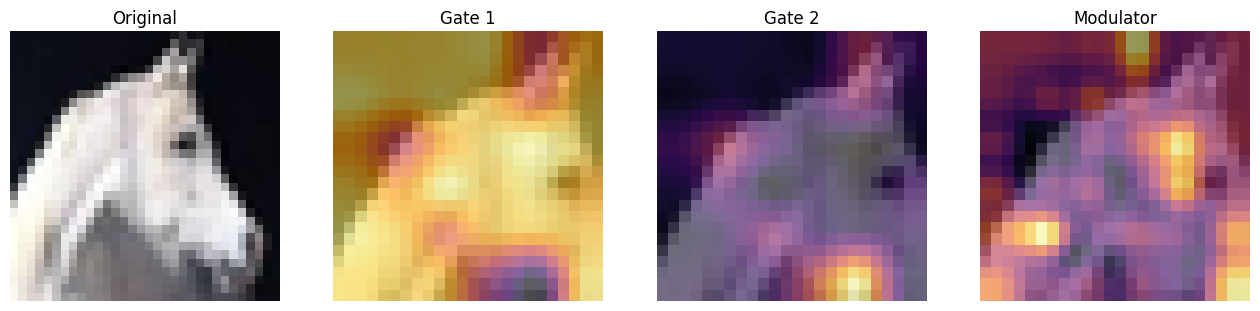``````40/40 [==============================] - 57s 724ms/step - loss: 2.3925 - accuracy: 0.1401 - val_loss: 2.2182 - val_accuracy: 0.1768
Epoch 2/25
40/40 [==============================] - 20s 483ms/step - loss: 2.0790 - accuracy: 0.2261 - val_loss: 2.2933 - val_accuracy: 0.1795
Epoch 3/25
40/40 [==============================] - 19s 479ms/step - loss: 2.0130 - accuracy: 0.2585 - val_loss: 2.6833 - val_accuracy: 0.2022
Epoch 4/25
40/40 [==============================] - 21s 507ms/step - loss: 1.8270 - accuracy: 0.3315 - val_loss: 1.9127 - val_accuracy: 0.3215
Epoch 5/25
40/40 [==============================] - 19s 475ms/step - loss: 1.6037 - accuracy: 0.4173 - val_loss: 1.7226 - val_accuracy: 0.3938
Epoch 6/25
40/40 [==============================] - 19s 476ms/step - loss: 1.4758 - accuracy: 0.4658 - val_loss: 1.5097 - val_accuracy: 0.4733
Epoch 7/25
40/40 [==============================] - 19s 476ms/step - loss: 1.3677 - accuracy: 0.5075 - val_loss: 1.4630 - val_accuracy: 0.4986
Epoch 8/25
40/40 [==============================] - 21s 508ms/step - loss: 1.2599 - accuracy: 0.5490 - val_loss: 1.2908 - val_accuracy: 0.5492
Epoch 9/25
40/40 [==============================] - 19s 478ms/step - loss: 1.1689 - accuracy: 0.5818 - val_loss: 1.2750 - val_accuracy: 0.5518
Epoch 10/25
40/40 [==============================] - 19s 476ms/step - loss: 1.0843 - accuracy: 0.6140 - val_loss: 1.1444 - val_accuracy: 0.6002
Epoch 11/25
39/40 [============================>.] - ETA: 0s - loss: 1.0040 - accuracy: 0.6453
``````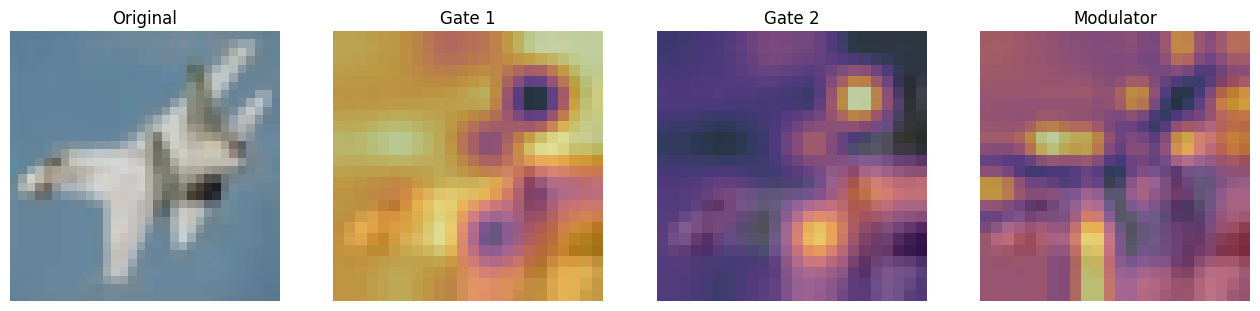``````40/40 [==============================] - 20s 489ms/step - loss: 1.0041 - accuracy: 0.6452 - val_loss: 1.1765 - val_accuracy: 0.5939
Epoch 12/25
40/40 [==============================] - 20s 480ms/step - loss: 0.9401 - accuracy: 0.6701 - val_loss: 1.1276 - val_accuracy: 0.6181
Epoch 13/25
40/40 [==============================] - 19s 480ms/step - loss: 0.8787 - accuracy: 0.6910 - val_loss: 0.9990 - val_accuracy: 0.6547
Epoch 14/25
40/40 [==============================] - 19s 479ms/step - loss: 0.8198 - accuracy: 0.7122 - val_loss: 1.0074 - val_accuracy: 0.6562
Epoch 15/25
40/40 [==============================] - 19s 480ms/step - loss: 0.7831 - accuracy: 0.7275 - val_loss: 0.9739 - val_accuracy: 0.6686
Epoch 16/25
40/40 [==============================] - 19s 478ms/step - loss: 0.7358 - accuracy: 0.7428 - val_loss: 0.9578 - val_accuracy: 0.6753
Epoch 17/25
40/40 [==============================] - 19s 478ms/step - loss: 0.7018 - accuracy: 0.7557 - val_loss: 0.9414 - val_accuracy: 0.6789
Epoch 18/25
40/40 [==============================] - 20s 480ms/step - loss: 0.6678 - accuracy: 0.7678 - val_loss: 0.9492 - val_accuracy: 0.6771
Epoch 19/25
40/40 [==============================] - 19s 476ms/step - loss: 0.6423 - accuracy: 0.7783 - val_loss: 0.9422 - val_accuracy: 0.6832
Epoch 20/25
40/40 [==============================] - 19s 479ms/step - loss: 0.6202 - accuracy: 0.7868 - val_loss: 0.9324 - val_accuracy: 0.6860
Epoch 21/25
40/40 [==============================] - ETA: 0s - loss: 0.6005 - accuracy: 0.7938
``````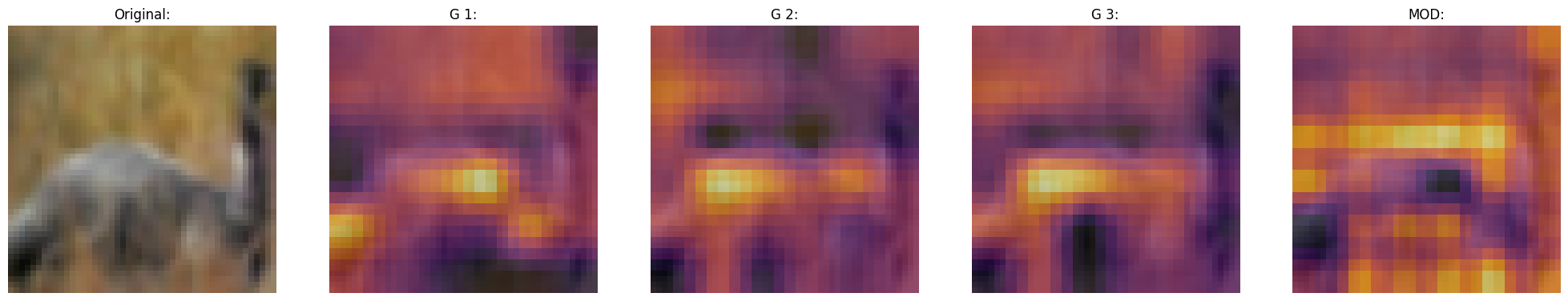``````40/40 [==============================] - 20s 488ms/step - loss: 0.6005 - accuracy: 0.7938 - val_loss: 0.9326 - val_accuracy: 0.6880
Epoch 22/25
40/40 [==============================] - 19s 478ms/step - loss: 0.5937 - accuracy: 0.7970 - val_loss: 0.9339 - val_accuracy: 0.6875
Epoch 23/25
40/40 [==============================] - 19s 478ms/step - loss: 0.5899 - accuracy: 0.7984 - val_loss: 0.9294 - val_accuracy: 0.6894
Epoch 24/25
40/40 [==============================] - 19s 478ms/step - loss: 0.5840 - accuracy: 0.8012 - val_loss: 0.9315 - val_accuracy: 0.6881
Epoch 25/25
40/40 [==============================] - 19s 478ms/step - loss: 0.5853 - accuracy: 0.7997 - val_loss: 0.9315 - val_accuracy: 0.6880
``````

## Plot loss and accuracy

``````plt.plot(history.history["loss"], label="loss")
plt.plot(history.history["val_loss"], label="val_loss")
plt.legend()
plt.show()

plt.plot(history.history["accuracy"], label="accuracy")
plt.plot(history.history["val_accuracy"], label="val_accuracy")
plt.legend()
plt.show()
``````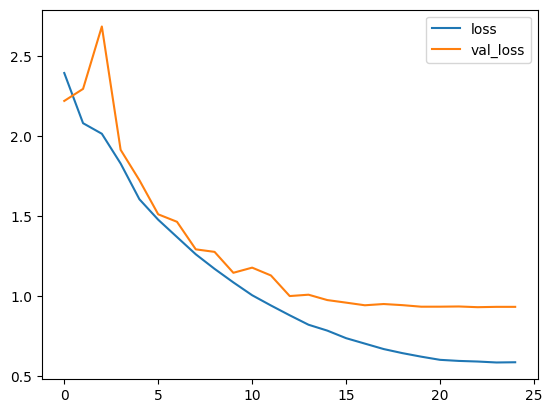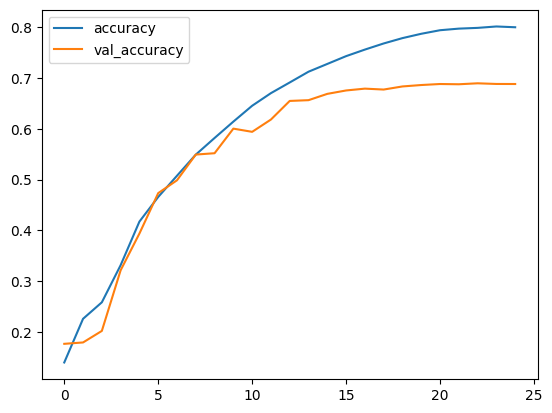## Test visualizations

Let's test our model on some test images and see how the gates look like.

``````test_images, test_labels = next(iter(test_ds))
_ = focal_mod_net(test_images)

# Take the mid layer for visualization
gates = focal_mod_net.basic_layers.blocks[-1].modulation.gates
gates = upsampler(gates)
modulator = focal_mod_net.basic_layers.blocks[-1].modulation.modulator
modulator = upsampler(modulator)

# Plot the test images with the gates and modulator overlayed.
for row in range(5):
display_grid(
test_images=test_images,
gates=gates,
modulator=modulator,
)
``````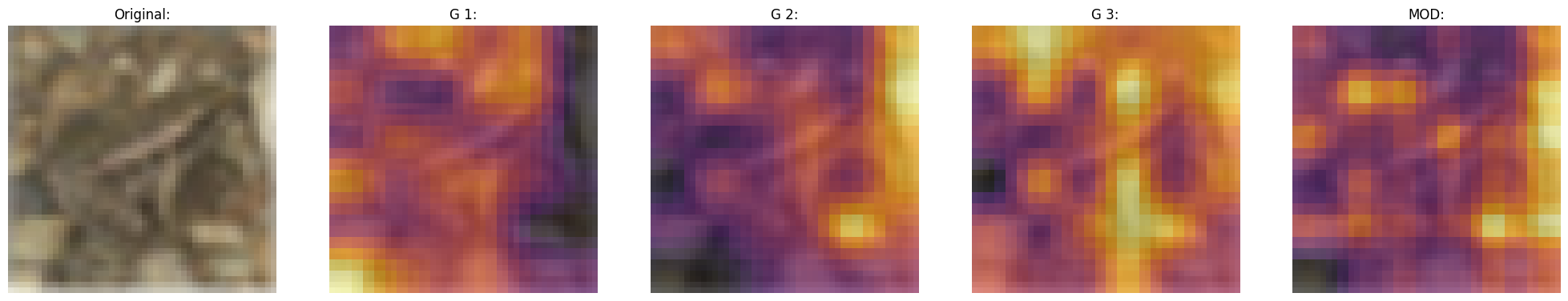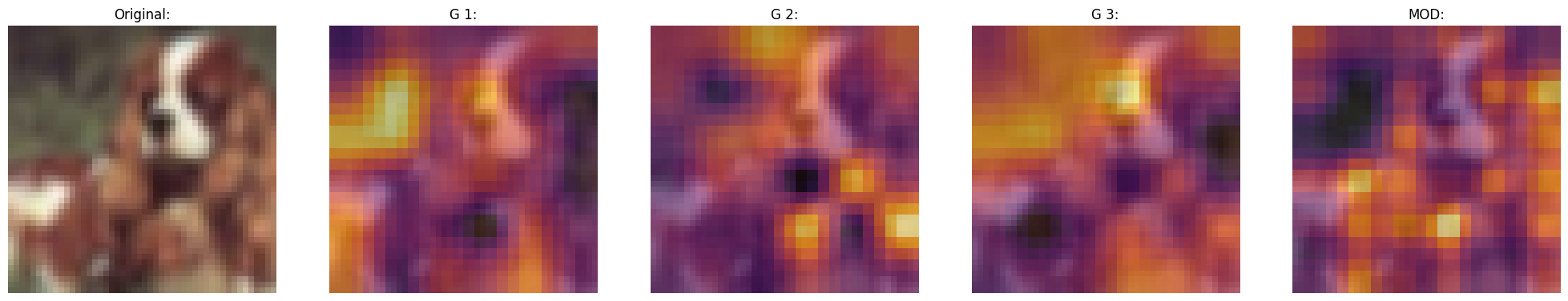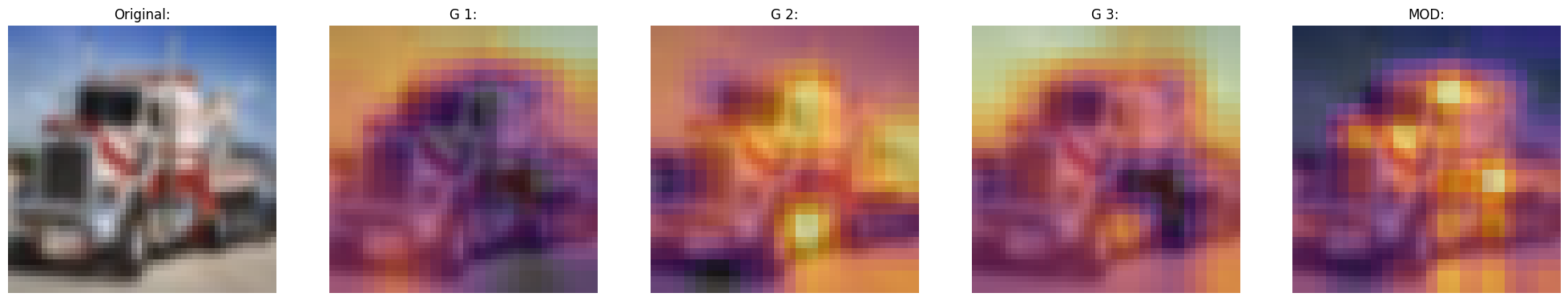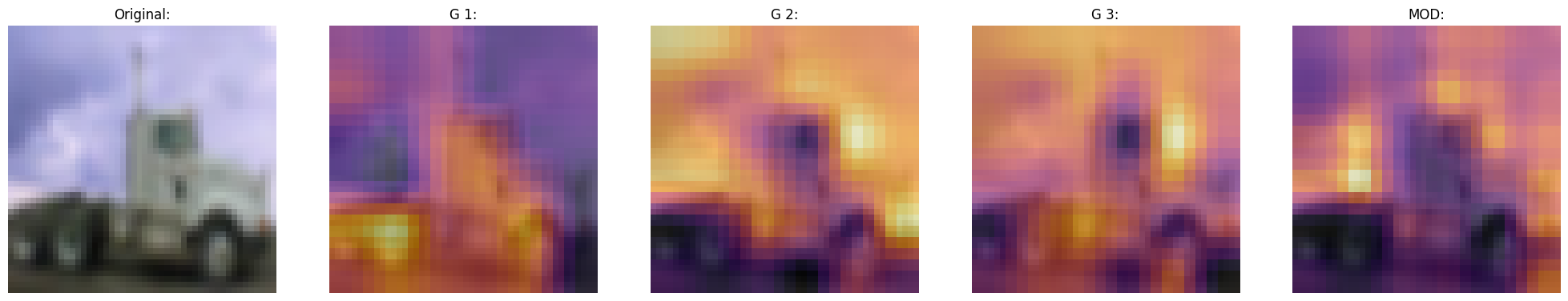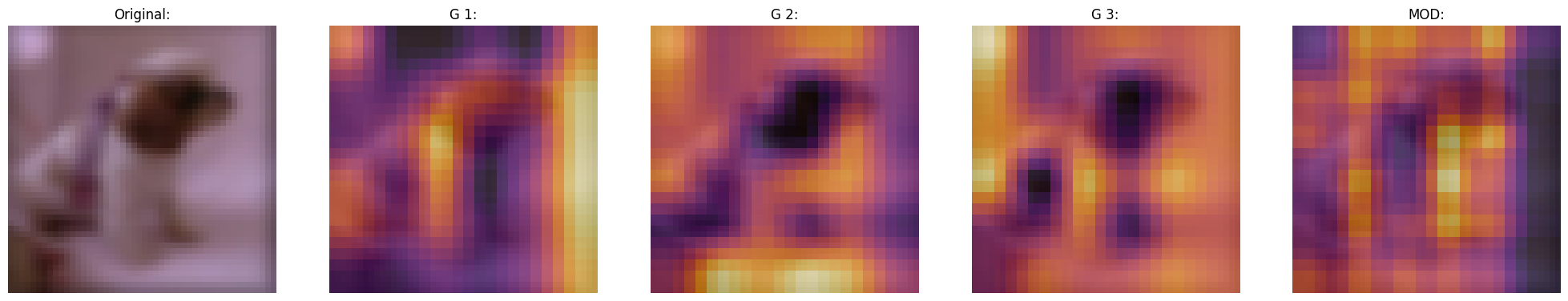## Conclusion

The proposed architecture, the Focal Modulation Network architecture is a mechanism that allows different parts of an image to interact with each other in a way that depends on the image itself. It works by first gathering different levels of context information around each part of the image (the "query token"), then using a gate to decide which context information is most relevant, and finally combining the chosen information in a simple but effective way.

This is meant as a replacement of Self-Attention mechanism from the Transformer architecture. The key feature that makes this research notable is not the conception of attention-less networks, but rather the introduction of a equally powerful architecture that is interpretable.

The authors also mention that they created a series of Focal Modulation Networks (FocalNets) that significantly outperform Self-Attention counterparts and with a fraction of parameters and pretraining data.

The FocalNets architecture has the potential to deliver impressive results and offers a simple implementation. Its promising performance and ease of use make it an attractive alternative to Self-Attention for researchers to explore in their own projects. It could potentially become widely adopted by the Deep Learning community in the near future.

## Acknowledgement

We would like to thank PyImageSearch for providing with a Colab Pro account, JarvisLabs.ai for GPU credits, and also Microsoft Research for providing an official implementation of their paper. We would also like to extend our gratitude to the first author of the paper Jianwei Yang who reviewed this tutorial extensively.